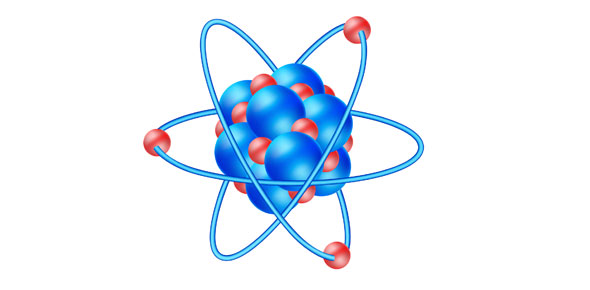12 Questions | Total Attempts: 132SettingsPls try out this quiz to test your understanding.

• 1.
The nucleus of an atom
• A.

Always contains protons and neutrons

• B.

Is neutral

• C.

Contains protons only

• D.

Usually contains protons and neutrons

• 2.
Which of the following statements about the atom is true?
• A.

No of electrons= no of protons

• B.

No of protons = no of neutrons

• C.

No of electrons = no of neutrons

• D.

No of neutrons = no of protons

• 3.
The neutron is a particle that has
• A.

A mass of 1g, which is the same as that of a proton

• B.

The same charge as the electron

• C.

A mass that is approximately that of the proton

• D.

The same charge as that of the proton

• 4.
The nucleon number of chlorine is 35 and its proton number is 17. It can therefore be deduced that the atom has
• A.

35 neutrons

• B.

18 neutrons

• C.

17 neutrons

• D.

35 protons and electrons

• 5.
All isotopes of an element contain
• A.

The same number of electrons as neutrons

• B.

The same number of protons as neutrons

• C.

The same number of neutrons

• D.

The same number of protons

• 6.
The structures of five atoms are given in the table below. Determine which pairs are isotopes. Atom No. of neutrons No. of electrons No. of protons P 16 15 15 Q 16 16 16 R 17 15 15 S 17 17 17 T 18 16 16
• A.

P & R

• B.

P & S

• C.

Q & R

• D.

Q & S

• 7.
The chlorine atom and ion are
• A.

Chemically identical

• B.

Have the same number of electrons

• C.

Isotopes

• D.

Have the same number of protons

• 8.
The atoms in the Periodic Table are arranged in terms of
• A.

Ascending proton numbers

• B.

Electrons lost

• C.

Descending proton numbers

• D.

Electrons gained

• 9.
The sulphur atom contains 16 electrons. It can be deduced that
• A.

It has 16 valence electrons

• B.

The atomic mass is 16

• C.

It is found in group 6

• D.

It is a metal

• 10.
Elements in the same group of the Periodic Table have the
• A.

Same number of electrons

• B.

Same number of valence electrons

• C.

The same number of electron shells

• D.

The same number of filled electron shells

• 11.
Valence electrons refer to those that
• A.

Are found in the first electron shell of the atom

• B.

Are given away by an atom to form an ion

• C.

Are taken in by the atom

• D.

Exist in the outermost shell of the atom

• 12.
Which of the following represents the electronic configuration of calcium (atomic number of Ca = 20)?
• A.

(2, 8, 10)

• B.

(2, 8, 8, 2)

• C.

(2, 10, 8)

• D.

(2, 18)

Related TopicsBack to top